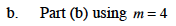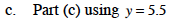### Home > MC1 > Chapter 4 > Lesson 4.3.4 > Problem4-123

4-123.
1. . Use the values given below for the lengths in the diagrams from problem 4-122 to find the total length of each segment. For example, if x were equal to 3 units in part (a) of problem 4-122, the line segment would be 3 + 1 + 3 = 7 units long. Homework Help ✎

1. Part (a) using x = 4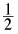2. Part (b) using m = 4

3. Part (c) using y = 5.5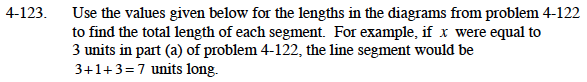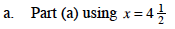Remember that the expression for part (a) is x + 1 + x.
If you put in the value for x and simplified the expression, what answer would you get?
${4\frac{1}{2}+1+4\frac{1}{2}=10}$